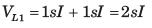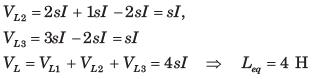Courses

# Test: Coupled Circuits Part - 2

## 10 Questions MCQ Test Networking Theory | Test: Coupled Circuits Part - 2

Description
This mock test of Test: Coupled Circuits Part - 2 for Electrical Engineering (EE) helps you for every Electrical Engineering (EE) entrance exam. This contains 10 Multiple Choice Questions for Electrical Engineering (EE) Test: Coupled Circuits Part - 2 (mcq) to study with solutions a complete question bank. The solved questions answers in this Test: Coupled Circuits Part - 2 quiz give you a good mix of easy questions and tough questions. Electrical Engineering (EE) students definitely take this Test: Coupled Circuits Part - 2 exercise for a better result in the exam. You can find other Test: Coupled Circuits Part - 2 extra questions, long questions & short questions for Electrical Engineering (EE) on EduRev as well by searching above.
QUESTION: 1

### In the circuit shown in fig. P1.9.11 if current i1 = 5 cos (500t - 20 0) mA and i2 = 20 cos (500t - 200) mA, the total energy stored in system at t = 0 is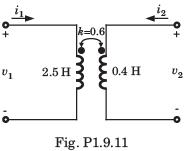Solution: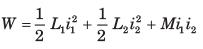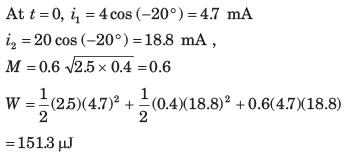QUESTION: 2

### Leq = ?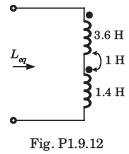Solution: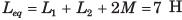QUESTION: 3

### Leq = ?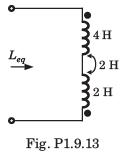Solution: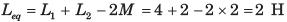QUESTION: 4

Leq = ?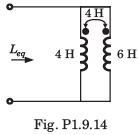Solution: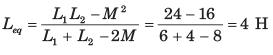QUESTION: 5

Leq = ?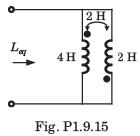Solution: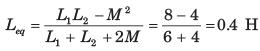QUESTION: 6

The equivalent inductance of a pair of a coupled inductor in various configuration are
(a) 7 H after series adding connection
(b) 1.8 H after series opposing connection
(c) 0.5 H after parallel connection with dotted terminal connected together.
The value of L1 ,L2 and M are

Solution: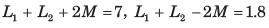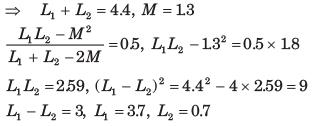QUESTION: 7

Leq = ?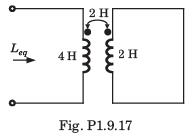Solution: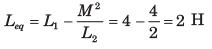QUESTION: 8

Leq = ?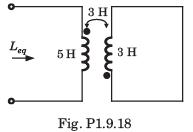Solution: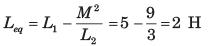QUESTION: 9

In the network of fig. P1.9.19 following terminal are connected together
(i) none (ii) A to B (iii) B to C (iv) A to C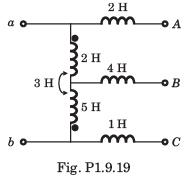The correct match for equivalent induction seen at terminal a - b is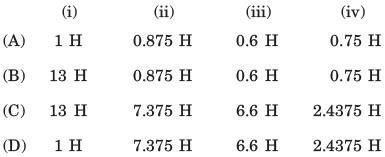Solution: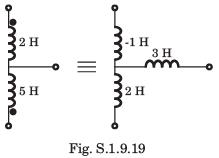QUESTION: 10

Leq =?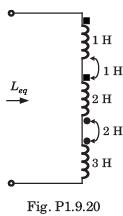Solution: